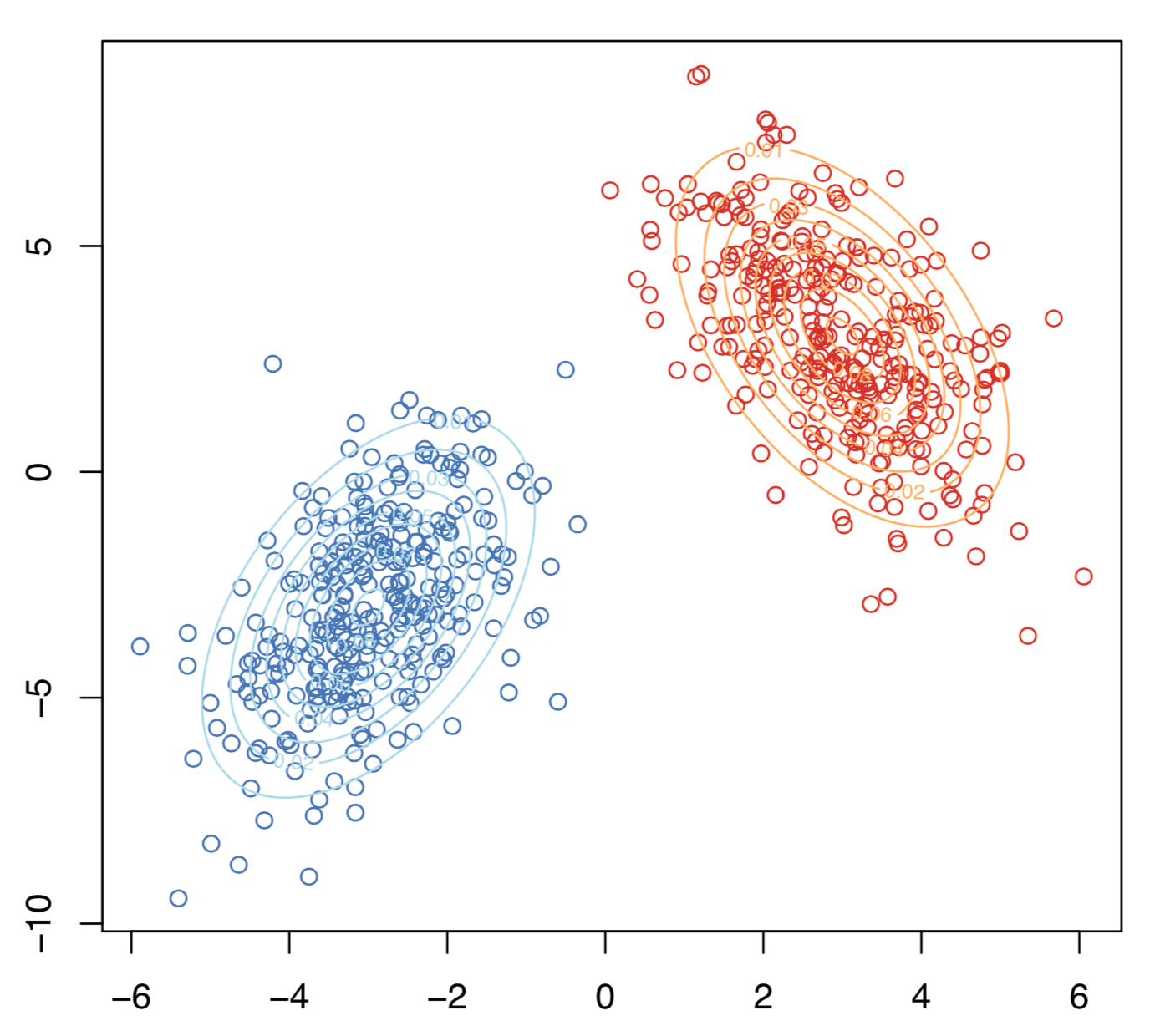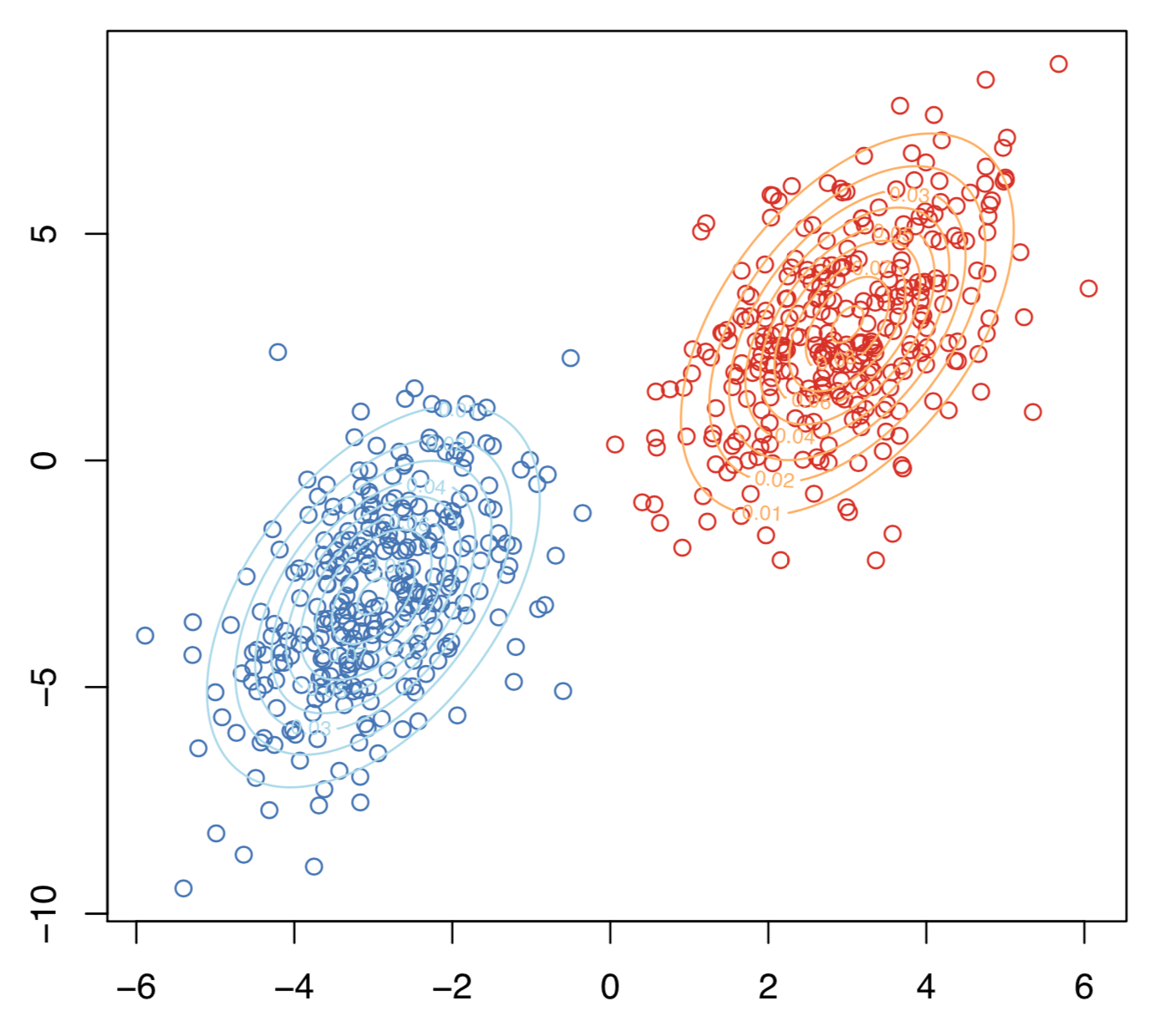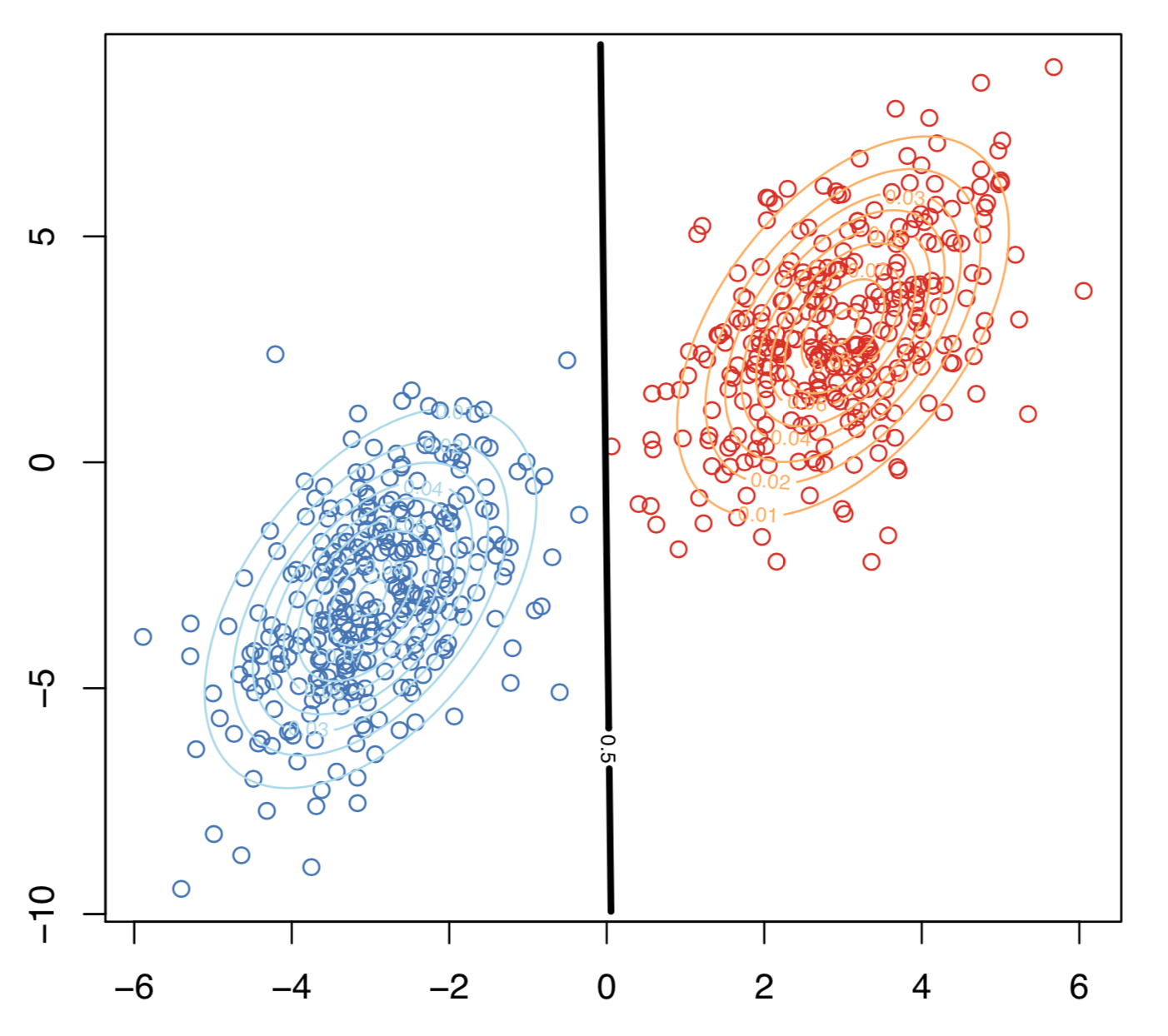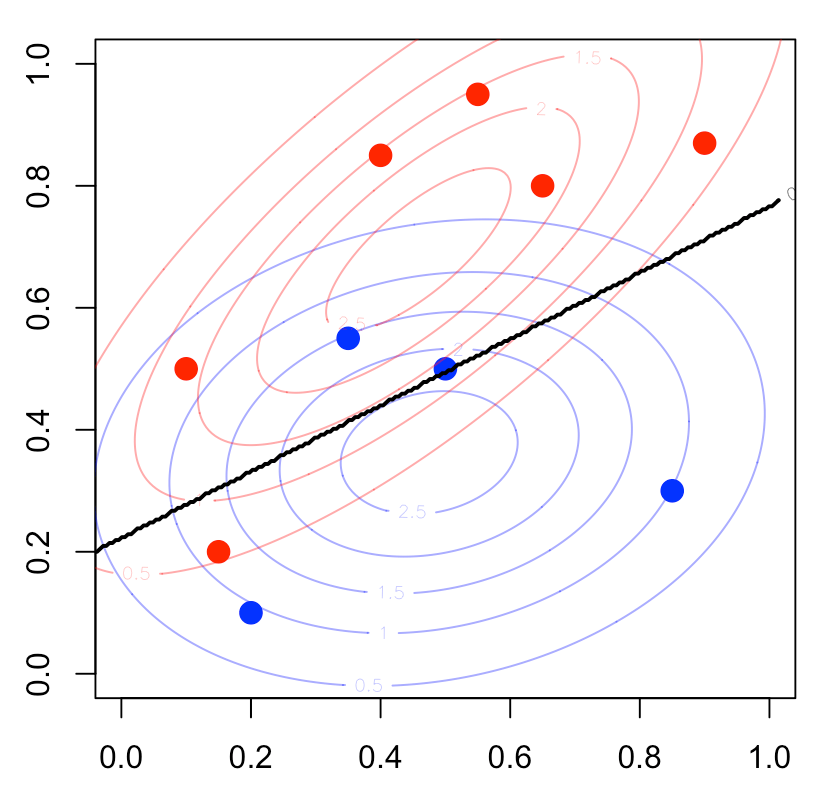Want to share your content on R-bloggers? click here if you have a blog, or here if you don't.

Eighth post of our series on classification from scratch. The latest one was on the SVM, and today, I want to get back on very old stuff, with here also a linear separation of the space, using Fisher’s linear discriminent analysis.

## Bayes (naive) classifier

Consider the follwing naive classification rule$$m^\star(\mathbf{x})=\text{argmin}_y\{\mathbb{P}[Y=y\vert\mathbf{X}=\mathbf{x}]\}$$or$$m^\star(\mathbf{x})=\text{argmin}_y\left\{\frac{\mathbb{P}[\mathbf{X}=\mathbf{x}\vert Y=y]}{\mathbb{P}[\mathbf{X}=\mathbf{x}]}\right\}$$(where $$\mathbb{P}[\mathbf{X}=\mathbf{x}]$$ is the density in the continuous case).

In the case where $$y$$ takes two values, that will be standard $$\{0,1\}$$ here, one can rewrite the later as$$m^\star(\mathbf{x})=\begin{cases}1\text{ if }\mathbb{E}(Y\vert \mathbf{X}=\mathbf{x})>\displaystyle{\frac{1}{2}}\\0\text{ otherwise}\end{cases}$$and the set$$\mathcal{D}_S =\left\{\mathbf{x},\mathbb{E}(Y\vert \mathbf{X}=\mathbf{x})=\frac{1}{2}\right\}$$is called the decision boundary.

Assume that$$\mathbf{X}\vert Y=0\sim\mathcal{N}(\mathbf{\mu}_0,\mathbf{\Sigma})$$and$$\mathbf{X}\vert Y=1\sim\mathcal{N}(\mathbf{\mu}_1,\mathbf{\Sigma})$$then explicit expressions can be derived.$$m^\star(\mathbf{x})=\begin{cases}1\text{ if }r_1^2< r_0^2+2\displaystyle{\log\frac{\mathbb{P}(Y=1)}{\mathbb{P}(Y=0)}+\log\frac{\vert\mathbf{\Sigma}_0\vert}{\vert\mathbf{\Sigma}_1\vert}}\\0\text{ otherwise}\end{cases}[/latex]where $r_y^2$ is the Manalahobis distance, [latex display="true"]r_y^2 = [\mathbf{X}-\mathbf{\mu}_y]^{\text{{T}}}\mathbf{\Sigma}_y^{-1}[\mathbf{X}-\mathbf{\mu}_y][/latex] Let [latex]\delta_y$$be defined as$$\delta_y(\mathbf{x})=-\frac{1}{2}\log\vert\mathbf{\Sigma}_y\vert-\frac{1}{2}[{\color{blue}{\mathbf{x}}}-\mathbf{\mu}_y]^{\text{{T}}}\mathbf{\Sigma}_y^{-1}[{\color{blue}{\mathbf{x}}}-\mathbf{\mu}_y]+\log\mathbb{P}(Y=y)$$the decision boundary of this classifier is $$\{\mathbf{x}\text{ such that }\delta_0(\mathbf{x})=\delta_1(\mathbf{x})\}$$which is quadratic in $${\color{blue}{\mathbf{x}}}$$. This is the quadratic discriminant analysis. This can be visualized bellow.The decision boundary is hereBut that can’t be the linear discriminant analysis, right? I mean, the frontier is not linear… Actually, in Fisher’s seminal paper, it was assumed that $$\mathbf{\Sigma}_0=\mathbf{\Sigma}_1$$.

In that case, actually, $$\delta_y(\mathbf{x})={\color{blue}{\mathbf{x}}}^{\text{T}}\mathbf{\Sigma}^{-1}\mathbf{\mu}_y-\frac{1}{2}\mathbf{\mu}_y^{\text{T}}\mathbf{\Sigma}^{-1}\mathbf{\mu}_y+\log\mathbb{P}(Y=y)$$ and the decision frontier is now linear in $${\color{blue}{\mathbf{x}}}$$. This is the linear discriminant analysis. This can be visualized bellowHere the two samples have the same variance matrix and the frontier is## Link with the logistic regression

Assume as previously that$$\mathbf{X}\vert Y=0\sim\mathcal{N}(\mathbf{\mu}_0,\mathbf{\Sigma})$$and$$\mathbf{X}\vert Y=1\sim\mathcal{N}(\mathbf{\mu}_1,\mathbf{\Sigma})$$then$$\log\frac{\mathbb{P}(Y=1\vert \mathbf{X}=\mathbf{x})}{\mathbb{P}(Y=0\vert \mathbf{X}=\mathbf{x})}$$is equal to $$\mathbf{x}^{\text{{T}}}\mathbf{\Sigma}^{-1}[\mathbf{\mu}_y]-\frac{1}{2}[\mathbf{\mu}_1-\mathbf{\mu}_0]^{\text{{T}}}\mathbf{\Sigma}^{-1}[\mathbf{\mu}_1-\mathbf{\mu}_0]+\log\frac{\mathbb{P}(Y=1)}{\mathbb{P}(Y=0)}$$which is linear in $$\mathbf{x}$$$$\log\frac{\mathbb{P}(Y=1\vert \mathbf{X}=\mathbf{x})}{\mathbb{P}(Y=0\vert \mathbf{X}=\mathbf{x})}=\mathbf{x}^{\text{{T}}}\mathbf{\beta}$$Hence, when each groups have Gaussian distributions with identical variance matrix, then LDA and the logistic regression lead to the same classification rule.

Observe furthermore that the slope is proportional to $$\mathbf{\Sigma}^{-1}[\mathbf{\mu}_1-\mathbf{\mu}_0]$$, as stated in Fisher’s article. But to obtain such a relationship, he observe that the ratio of between and within variances (in the two groups) was$$\frac{\text{variance between}}{\text{variance within}}=\frac{[\mathbf{\omega}\mathbf{\mu}_1-\mathbf{\omega}\mathbf{\mu}_0]^2}{\mathbf{\omega}^{\text{T}}\mathbf{\Sigma}_1\mathbf{\omega}+\mathbf{\omega}^{\text{T}}\mathbf{\Sigma}_0\mathbf{\omega}}$$which is maximal when $$\mathbf{\omega}$$ is proportional to $$\mathbf{\Sigma}^{-1}[\mathbf{\mu}_1-\mathbf{\mu}_0]$$, when $$\mathbf{\Sigma}_0=\mathbf{\Sigma}_1$$.

## Homebrew linear discriminant analysis

To compute vector $$\mathbf{\omega}$$

m0 = apply(myocarde[myocarde$PRONO=="0",1:7],2,mean) m1 = apply(myocarde[myocarde$PRONO=="1",1:7],2,mean)
Sigma = var(myocarde[,1:7])
omega = solve(Sigma)%*%(m1-m0)
omega
[,1]
FRCAR -0.012909708542
INCAR  1.088582058796
INSYS -0.019390084344
PRDIA -0.025817110020
PAPUL  0.020441287970
PVENT -0.038298291091
REPUL -0.001371677757

For the constant – in the equation $$\omega^T\mathbf{x}+b=0$$ – if we have equiprobable probabilities, use

b = (t(m1)%*%solve(Sigma)%*%m1-t(m0)%*%solve(Sigma)%*%m0)/2

## Application (on the small dataset)

In order to visualize what’s going on, consider the small dataset, with only two covariates,

x = c(.4,.55,.65,.9,.1,.35,.5,.15,.2,.85)
y = c(.85,.95,.8,.87,.5,.55,.5,.2,.1,.3)
z = c(1,1,1,1,1,0,0,1,0,0)
df = data.frame(x1=x,x2=y,y=as.factor(z))
m0 = apply(df[df$y=="0",1:2],2,mean) m1 = apply(df[df$y=="1",1:2],2,mean)
Sigma = var(df[,1:2])
omega = solve(Sigma)%*%(m1-m0)
omega
[,1]
x1 -2.640613174
x2  4.858705676Using R regular function, we get

library(MASS)
fit_lda = lda(y ~x1+x2 , data=df)
fit_lda

Coefficients of linear discriminants:
LD1
x1 -2.588389554
x2  4.762614663

which is the same coefficient as the one we got with our own code. For the constant, use

b = (t(m1)%*%solve(Sigma)%*%m1-t(m0)%*%solve(Sigma)%*%m0)/2

If we plot it, we get the red straight line

plot(df$x1,df$x2,pch=c(1,19)[1+(df$y=="1")]) abline(a=b/omega,b=-omega/omega,col="red")As we can see (with the blue points), our red line intersects the middle of the segment of the two barycenters points(m0["x1"],m0["x2"],pch=4) points(m1["x1"],m1["x2"],pch=4) segments(m0["x1"],m0["x2"],m1["x1"],m1["x2"],col="blue") points(.5*m0["x1"]+.5*m1["x1"],.5*m0["x2"]+.5*m1["x2"],col="blue",pch=19) Of course, we can also use R function predlda = function(x,y) predict(fit_lda, data.frame(x1=x,x2=y))$class==1
vv=outer(vu,vu,predlda)
contour(vu,vu,vv,add=TRUE,lwd=2,levels = .5)One can also consider the quadratic discriminent analysis since it might be difficult to argue that $$\mathbf{\Sigma}_0=\mathbf{\Sigma}_1$$

fit_qda = qda(y ~x1+x2 , data=df)

The separation curve is here

plot(df$x1,df$x2,pch=19,
col=c("blue","red")[1+(df$y=="1")]) predqda=function(x,y) predict(fit_qda, data.frame(x1=x,x2=y))$class==1
vv=outer(vu,vu,predlda)
contour(vu,vu,vv,add=TRUE,lwd=2,levels = .5)